Explore

Exercises

# Fractions 4

Students are going to take a deeper dive into fractions in this unit! Learners will apply previous understanding of finding equivalent fractions, and converting between fractions and mixed numbers to work with fractions in more complex ways. Students will continue to use visual models to learn and practice adding, subtracting, multiplying and dividing fractions.
This lesson includes 5 printable learning activities.

## Adding Fractions with Unlike Denominators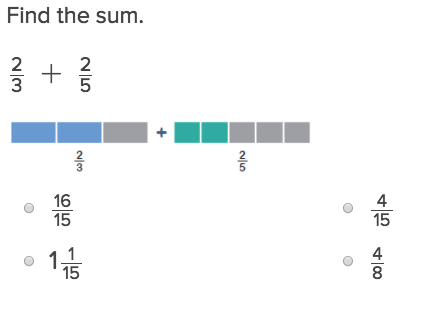Adding Fractions with Unlike Denominators will help students practice this key fifth grade skill.

## Adding Mixed Fractions with Like DenominatorsStudents will be able to add mixed number fractions after they learn how to make each number have like denominators.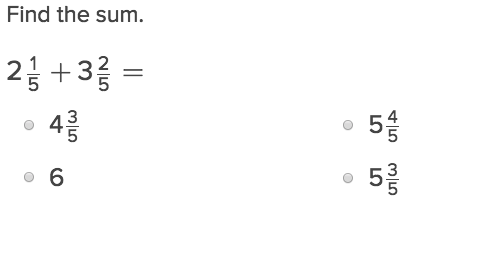Adding Mixed Number Fractions will help students practice this key fifth grade skill.

## City Fraction Subtraction with Like Denominators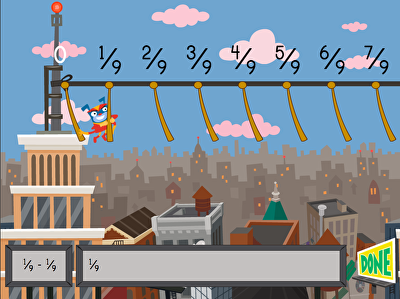Let's go on an adventure in the jungle! Floyd is ready to swing across the city but needs help answering fractions. Using a number line to visually see addition at work, kids answer fraction subtraction equations to help Floyd move backward in this city-themed game. Typically a challenging skill to master, kids will get lots of practice using the number line model to tackle fractions.

## Subtracting Fractions with the Same Denominator 2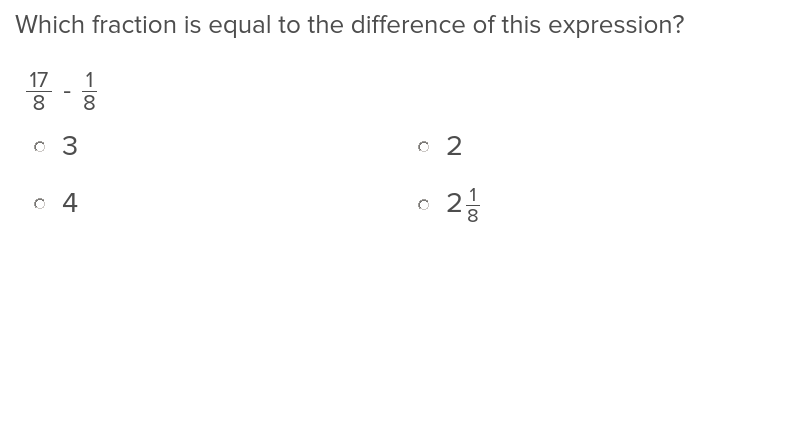Before students can master subtracting fractions, they’ll want to use this exercise to learn how to reach like denominators.

## Subtracting Fractions with Unlike Denominators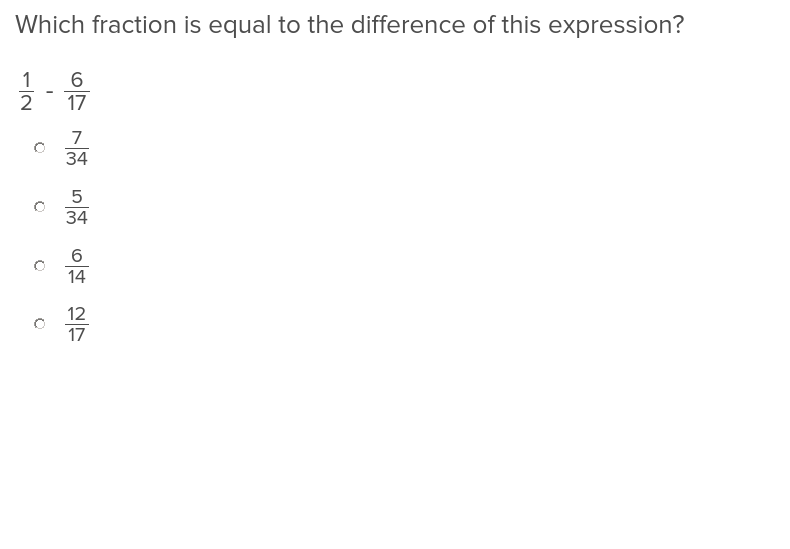Show students how to complete a fraction subtraction problem while working with unlike denominators with this easy to use exercise.

## Subtraction of Mixed Numbers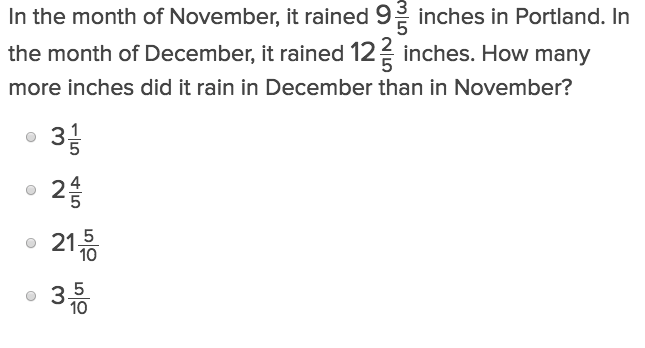Subtraction of Mixed Numbers will help students practice this key fifth grade skill.

## Addition and Subtraction of Mixed Numbers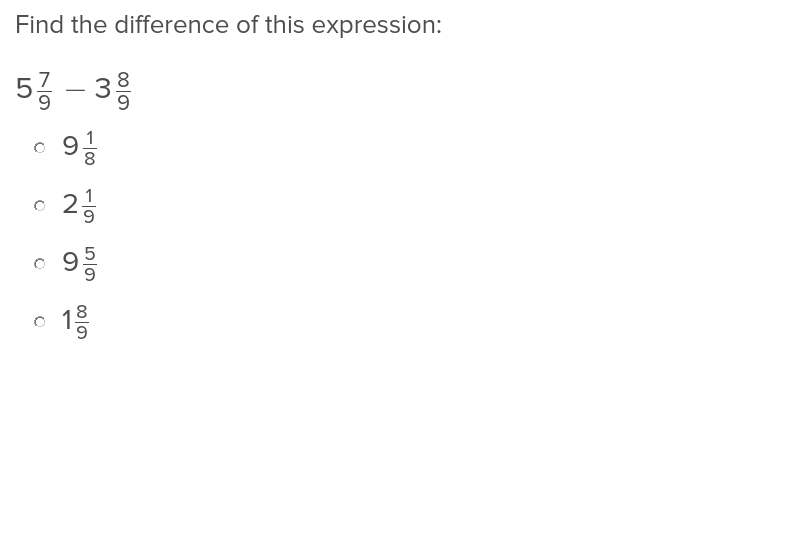Teach students how to add and subtract mixed numbers by assigning this straightforward Education.com exercise.

## Multiplication and Unit Fractions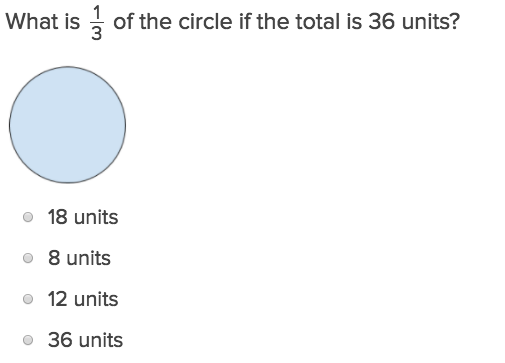Teach your class how to work through multiplying unit fractions with just a few clicks of the mouse using this exercise.

## Multiplying Fractions by Whole NumbersMultiplying Fractions by Whole Numbers will help students practice this key fifth grade skill.

## Multiplying Mixed Number Fractions and Arrays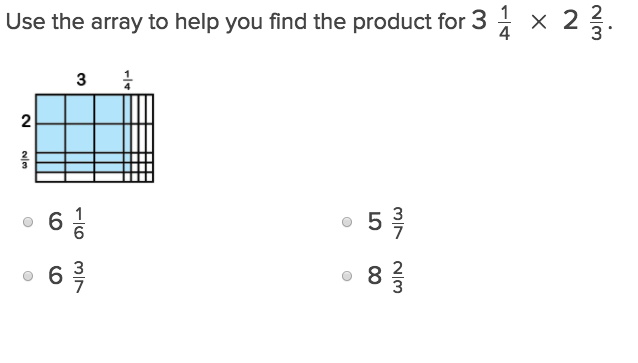Multiplying Mixed Number Fractions and Arrays will help students practice this key fifth grade skill.

## Multiplication of Fractions and Scaling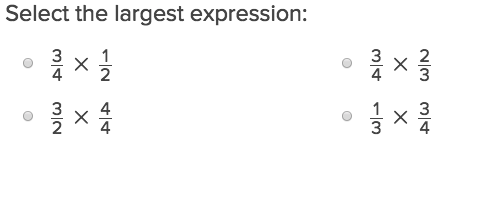Students who complete this exercise will understand how to scale up their newfound ability in multiplying fractions.

## Interpret Multiplication of Fractions as Scaling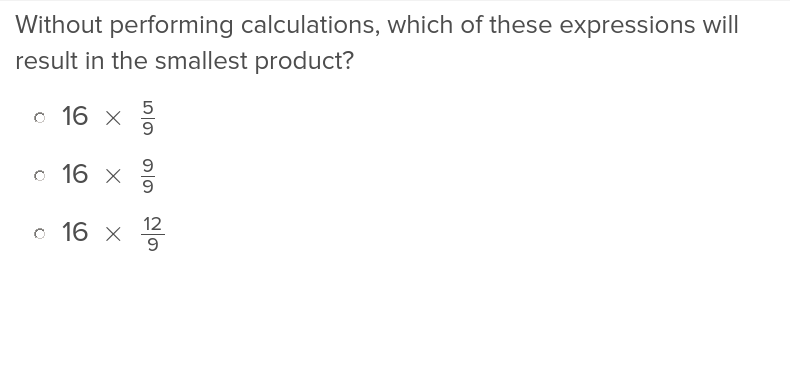Introduce your fifth graders to multiplying by fractions with these exercises that help them understand the effect of numerators and denominators of equations.

## Division with Unit FractionsDivision with Unit Fractions will help students practice this key fifth grade skill.

Create new collection

0

### New Collection>

0 items

What could we do to improve Education.com?